# Thevenin-Norton Equivalencies

## Chapter 10 - DC Network Analysis

Since Thevenin’s and Norton’s Theorems are two equally valid methods of reducing a complex network down to something simpler to analyze, there must be some way to convert a Thevenin equivalent circuit to a Norton equivalent circuit, and vice versa (just what you were dying to know, right?). Well, the procedure is very simple.

### Thevenin Resistance and Norton Resistance

You may have noticed that the procedure for calculating Thevenin resistance is identical to the procedure for calculating Norton resistance: remove all power sources and determine resistance between the open load connection points. As such, Thevenin and Norton resistances for the same original network must be equal. Using the example circuits from the last two sections, we can see that the two resistances are indeed equal: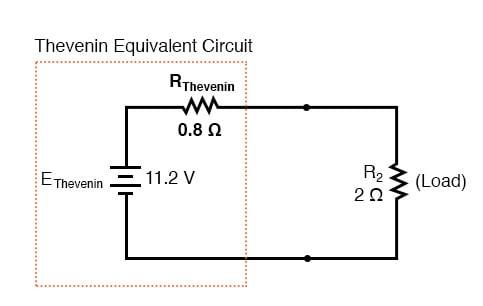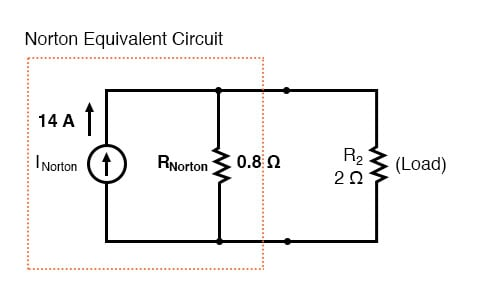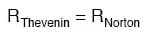Considering the fact that both Thevenin and Norton equivalent circuits are intended to behave the same as the original network in supplying voltage and current to the load resistor (as seen from the perspective of the load connection points), these two equivalent circuits, having been derived from the same original network should behave identically.

This means that both Thevenin and Norton equivalent circuits should produce the same voltage across the load terminals with no load resistor attached. With the Thevenin equivalent, the open-circuited voltage would be equal to the Thevenin source voltage (no circuit current present to drop voltage across the series resistor), which is 11.2 volts in this case. With the Norton equivalent circuit, all 14 amps from the Norton current source would have to flow through the 0.8 Ω Norton resistance, producing the exact same voltage, 11.2 volts (E=IR). Thus, we can say that the Thevenin voltage is equal to the Norton current times the Norton resistance: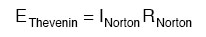So, if we wanted to convert a Norton equivalent circuit to a Thevenin equivalent circuit, we could use the same resistance and calculate the Thevenin voltage with Ohm’s Law.

Conversely, both Thevenin and Norton equivalent circuits should generate the same amount of current through a short circuit across the load terminals. With the Norton equivalent, the short-circuit current would be exactly equal to the Norton source current, which is 14 amps in this case. With the Thevenin equivalent, all 11.2 volts would be applied across the 0.8 Ω Thevenin resistance, producing the exact same current through the short, 14 amps (I=E/R). Thus, we can say that the Norton current is equal to the Thevenin voltage divided by the Thevenin resistance: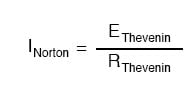This equivalence between Thevenin and Norton circuits can be a useful tool in itself, as we shall see in the next section.

REVIEW:

• Thevenin and Norton’s resistances are equal.
• Thevenin voltage is equal to Norton's current times Norton resistance.
• Norton current is equal to Thevenin voltage divided by Thevenin resistance.

RELATED WORKSHEETS:

Published under the terms and conditions of the Design Science License
1 Comment•myxie June 25, 2020

The equality under the first drawing is incorrect. Both sides should be R subscript something.

Like.